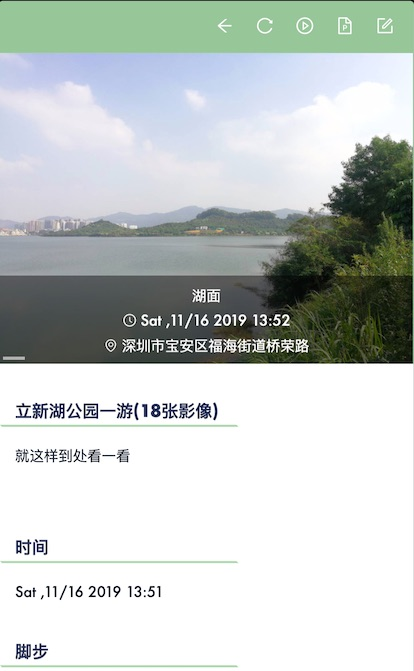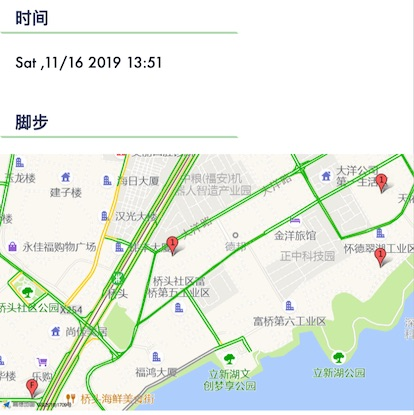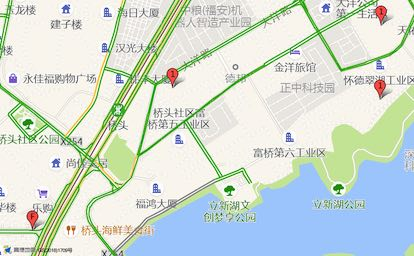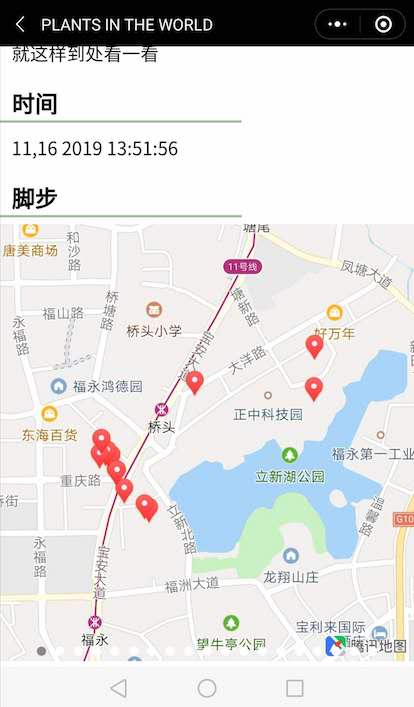### 我最近在做什么### 在有限的范围内容纳全部的坐标点

• 缩放级别，腾讯谓之 scale，高德称以 zoom
• 四个坐标点，其依次连接而形成的梯形（想一想，为什么是梯形？）恰如其分地将全部的坐标点融入梯形当中
• 实际的横纵距离
• “地理/地图”分辨率

``````interface Point {
longitude: number
latitude: number
}

type PointSet = Point[]
``````

``````interface Edge<X extends number, Y extends number> {
longitude: X
latitude: Y
}
``````

``````interface Trapezium {
T: number
R: number
B: number
L: number
}
``````
``````function getTRBL(points) {
let L = 135.083333 // maximum left
let R = 73.55 // minimal right
let B = 53.55 // maximum bottom
let T = 3.85 // minimal top
for (const p of points) {
const { longitude, latitude } = p
if (longitude < L) L = longitude
if (longitude > R) R = longitude
if (latitude < B) B = latitude
if (latitude > T) T = latitude
}
/**@type  Trapezium*/
return { T, R, B, L }
}
``````

• 在纵线上，也就是经线（Longitude Line），一个纬度的变化会引起 `111` km 的地表位移
• 在横线上，也就是纬线（Latitude Line），一个经度的变化所能引起的地表位移是“纬度”的函，它是 `111 * cos(la) ` km。
``````function distance(l, r, t, b) {
return [
(r - l) * 111 * Math.cos(b * Math.PI / 180 ),
(t - b) * 111
]
}
``````

``````// 单位是 cm / px
const resolution = [实际距离（厘米）] / [像素数量]
``````

``````const dX = (R - L) * 111 * Math.cos(B * Math.PI / 180)
const resoX = dX * 100000 / 414
``````

``````const dY = (T - B) * 111
const resoY = dY * 100000 / 360
``````

``````[
{ "zoom": 3, "reso": 1747785 },
{ "zoom": 4, "reso": 859400 },
{ "zoom": 5, "reso": 423048 },
{ "zoom": 6, "reso": 212032 },
{ "zoom": 7, "reso": 105777 },
{ "zoom": 8, "reso": 52829 },
{ "zoom": 9, "reso": 26399 },
{ "zoom": 10, "reso": 13196 },
{ "zoom": 11, "reso": 6597 },
{ "zoom": 12, "reso": 3267 },
{ "zoom": 13, "reso": 1634 },
{ "zoom": 14, "reso": 817 },
{ "zoom": 15, "reso": 408 },
{ "zoom": 16, "reso": 204 },
{ "zoom": 17, "reso": 102 },
{ "zoom": 18, "reso": 51 },
{ "zoom": 19, "reso": 26 },
{ "zoom": 20, "reso": 0 }
]
``````

``````function getZoom(cmPerPx) {
for (let i = 0, l = relationOfZoomAndReso.length; i < l; i++) {
const { zoom, reso } = relationOfZoomAndReso[i]
if (reso < cmPerPx) {
return zoom - 1
}
}
return 11
}
``````

### 计算中心点

``````const center = { longitude: (T + B) / 2, latitude: (R + L) / 2 }
``````

### 调用地图接口及效果

``````<map setting="{{mapSettings}}" markers="{{markers}}" />
``````
``````Page({
// ...
data: {
mapSettings: {
scale: 14
},
markers: [...]
}
// ...
})
``````